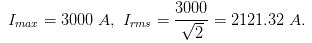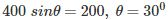# MCQs on Power Electronics

##### Page 1 of 10. Go to page 1 2 3 4 5 6 7 8 9 10
01․ The typical value of SCR for modern alternator is
1.5.
0.5.
1.0.
1.2.

Always the typical value of SCR for modern alternator is 0.5.

02․ An SCR has half cycle surge current rating of 3000 A for 50 Hz supply. One cycle surge current will be
1500 A.
6000 A.
2121.32 A.
4242.64 A.03․ An SCR is considered to be a semi controlled device because
it can be turned OFF but not ON with a gate pulse.
it conducts only during one half cycle of an alternating current wave.
it can be turned ON but not OFF with a gate pulse.
it can be turned ON only during one half cycle of an AC.

During positive half cycle SCR is in forward blocking mode. By applying gate pulse the SCR can be turned ON during forward blocking mode. But SCR can not be turned OFF by applying gate pulse. It automatically turns off when anode current is below the Holding current. That is why it is called semi controlled rectifier.

04․ A single phase one pulse controlled circuit has a resistance R and counter emf E load 400 sin(314 t) as the source voltage. For a load counter emf of 200 V, the range of firing angle control is
30° to 150°.
30° to 180°.
60° to 120°.
60° to 180°.So, control range is θ to ( π- θ ) i.e. 30° to 150°.

05․ A single phase full bridge inverter can operated in load commutation mode in case load consist of
RL.
RLC underdamped.
RLC overdamped.
RLC critically damped.

In this case reading current will flow in circuit and it will became zero so thyristor will be load commutated .

06․ A step up chopper has input voltage 110 V and output voltage 150 V. The value of duty cycle is
0.32.
0.67.
0.45.
0.27.

For step-up chopper, E0=Edc/(1-α), E0 = output voltage and Edc = output voltage,α = 1-(110/150) ⇒ 0.26 where α = duty cycle. recheck

07․ Which statement is true for latching current ?
It is related to turn off process of the device.
It is related to conduction process of device.
It is related to turn on process of the device.
Both C and D.

Latching current is the minimum required anode current to turn on SCR.

08․ If holding current of a thyristor is 2 mA then latching current should be
0.01 A.
0.002 A.
0.009 A.
0.004 A.

Generally latching current is 2 or 3 times of holding current.

09․ In reverse blocking mode of a thyristor
junction J2 is in reverse bias and J1, J3 is in forward bias.
junction J3 is in forward bias and J1, J2 in reverse bias.
junction J1, J3 is in reverse bias and J2 is in forward bias.
junction J1 and J2 is in forward bias and J3 is in reverse bias.

In reverse blocking mode J1 and J2 is in reverse bias and J2 is in forward bias.

10․ In forward blocking mode of a thyristor
junction J2 is in reverse bias and J1, J3 is in forward bias.
junction J3 is in forward bias and J1, J2 is in reverse bias.
Junction J1, J3 is in reverse bias and J2 is in forward bias.
Junction J1 and J2 is in forward bias and J3 is in reverse bias.

In forward blocking mode junction J2 acts in reverse bias whereas J1 and J3 act in forward bias.

123>>>